# DATEDIF Function

Calculates the number of days, months, or years between two dates

## What is the DATEDIF Function?

The DATEDIF Function in Excel is categorized under Excel Date/Time functions. As a financial analyst, we may require the number of days, months, or years between two dates. The DATEDIF function helps us calculate the difference.

### Formula

=DATEDIF(start_date,end_date,unit)

The DATEDIF function includes the following arguments:

1. Start_date – This is a required argument. As the name suggests, it is the initial date of the period.
2. End_date – This is also a required argument. It represents the last, or ending, date of the period.
3. Unit – The time unit in which we want the information.

UnitReturns
“Y”Difference in complete years. The function returns the number of complete years in the period.
“M”Difference in complete months. The function returns the number of complete months in the period.
“D”Difference in complete Days. The function returns the number of complete Days in the period.
“MD”It calculates the difference between the days in start_date and end_date. The months and years of the dates are ignored here.
“YM”It calculates the difference between the months in start_date and end_date. The days and years of the dates are ignored here.

Interestingly, DATEDIF is a hidden function in Excel. As seen below, Excel doesn’t list this function when we type =DATE in a cell. Hence, we need to understand thoroughly the syntax of this function.

### How to use the DATEDIF Function in Excel?

To understand the uses of this function, let’s consider a few examples:

#### Example 1

Let’s say we wish to find the number of days between May 10, 2015 and July 10, 2017. The formula to use would be: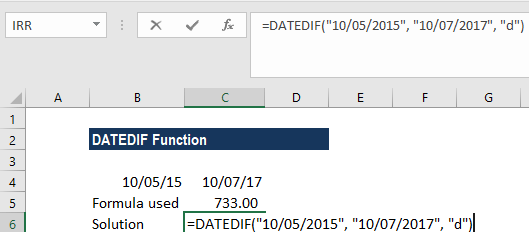We can, on a similar basis, provide cell references. For example, the following formula counts the number of days between the dates in cells A1 and B1: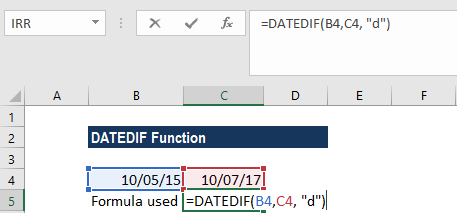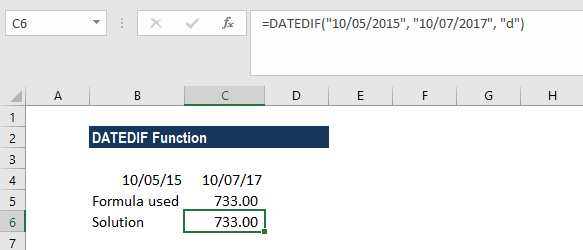As we are aware that Excel stores each date as a serial number beginning with January 1, 1900, so we can put numbers corresponding to the dates directly in the formula. For example, =DATEDIF(42134, 42205, “m”)

However, this method is not fully reliable as date numbering varies with different computer operating systems.

#### Example 2

Suppose we receive a list of debtors with outstanding debts. We wish to calculate the number of days from the day they were required to pay until today, that is, July 31, 2017.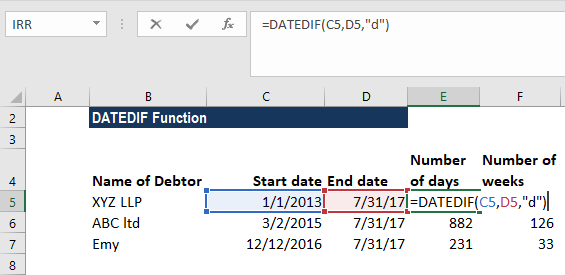The solution we get is: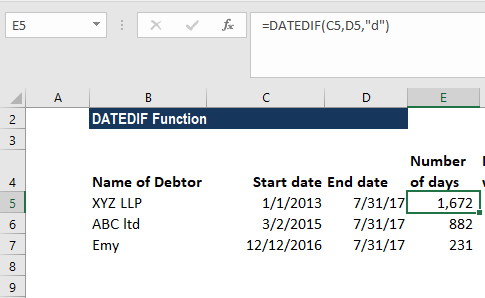Suppose you want the difference in weeks. To find out how many weeks there are between two dates, we can use the DATEDIF function with “D” unit to return the difference in days, and then divide the result by 7.

The formula to be used is: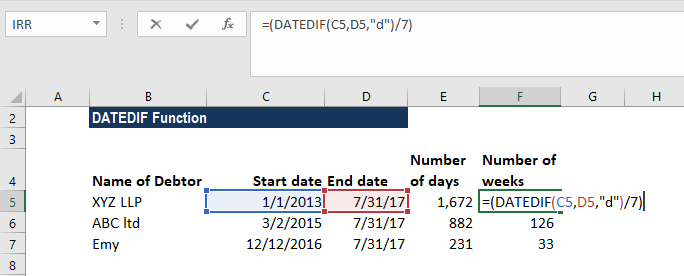The solution we get is in weeks: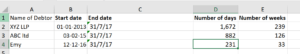If we want, we can wrap the DATEDIF formula in the ROUNDDOWN function, which always rounds the number towards zero:

=ROUNDDOWN((DATEDIF(B2, A2, “d”) / 7), 0)

#### Example 3

To count the number of whole months between dates, DATEDIF function with “M” unit can be used. The formula =DATEDIF(start_date, end_date, “m”) compares the dates in A2 (start date) and B2 (end date) and returns the difference in months:

Suppose we are given the following dates: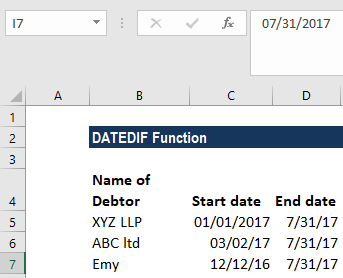The formula used is: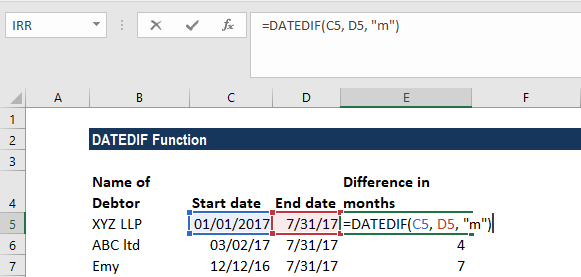The solution we get is: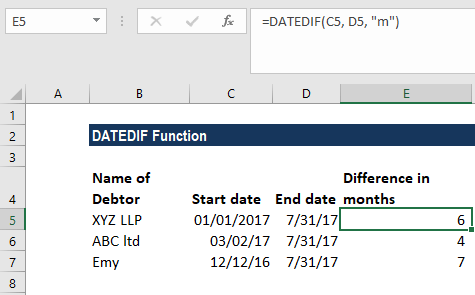If you wish to ignore years, the formula to use would be =DATEDIF(start_date, end_date, “ym”)

The data given are: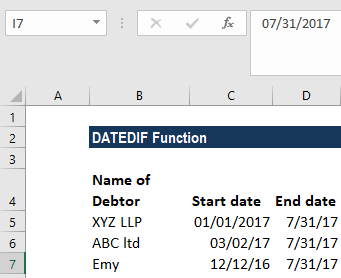The formula used is: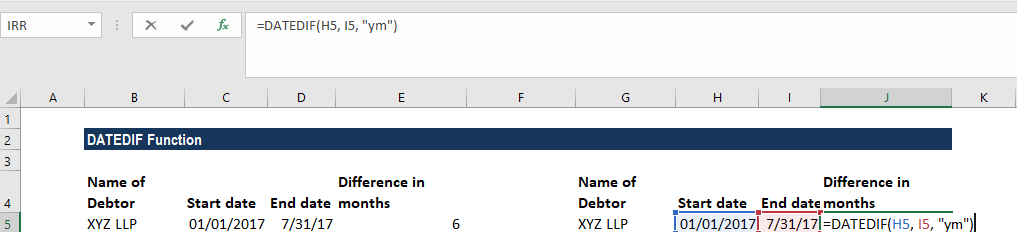The solution we get is: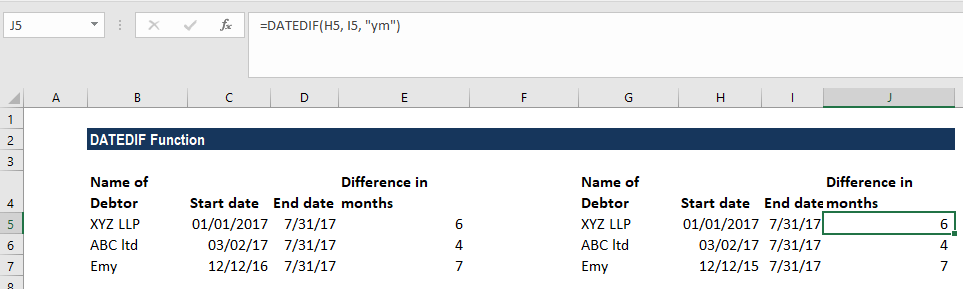#### Example 4

Suppose a flower store is required to send flowers to its customers on an anniversary date. We wish to calculate the next anniversary date. The data given are: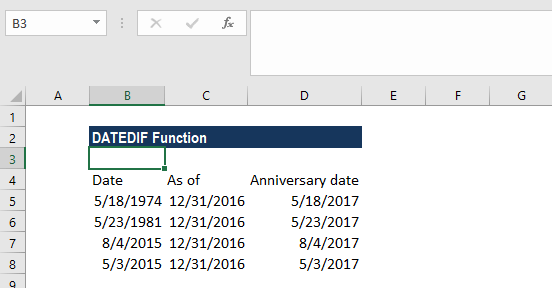The formula to be used is: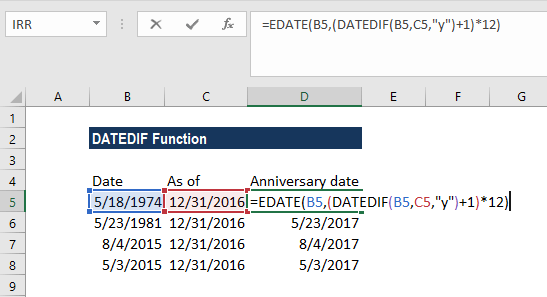The solution we get is: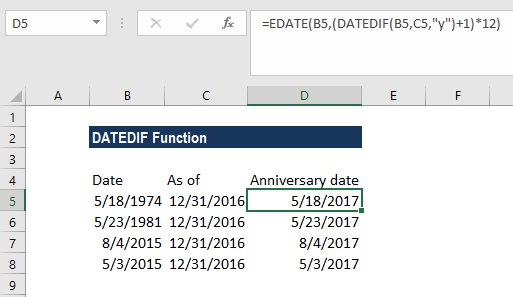### Notes about the DATEDIF Function in Excel

1. #NUM! error – Occurs when the start_date is greater than the end_date.
2. #VALUE! Error – Occurs when Excel cannot recognize the date given, i.e., when the date argument is invalid.

Thanks for reading CFI’s guide to important Excel functions! By taking the time to learn and master these functions, you’ll significantly speed up your financial analysis. To learn more, check out these additional CFI resources:

• Excel Functions for Finance